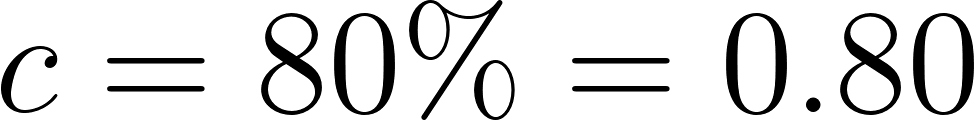×
Get Full Access to Probability And Statistical Inference - 9 Edition - Chapter 7.4 - Problem 14e
Get Full Access to Probability And Statistical Inference - 9 Edition - Chapter 7.4 - Problem 14e

×

# If Y1/n and Y2/n are the respective independent relativeISBN: 9780321923271 41

## Solution for problem 14E Chapter 7.4

Probability and Statistical Inference | 9th Edition

• Textbook Solutions
• 2901 Step-by-step solutions solved by professors and subject experts
• Get 24/7 help from StudySoup virtual teaching assistantsProbability and Statistical Inference | 9th Edition

4 5 1 401 Reviews
20
3
Problem 14E

If $$Y_{1} / n$$ and $$Y_{2} / n$$ are the respective independent relative frequencies of success associated with the two binomial distributions $$b\left(n, p_{1}\right)$$ and $$b\left(n, p_{2}\right)$$, compute $$n$$ such that the approximate probability that the random interval $$\left(Y_{1} / n-Y_{2} / n\right) \pm 0.05$$ covers $$p_{1}-p_{2}$$ is at least $$0.80$$. Hint: Take $$p_{1}^{*}=p_{2}^{*}=1 / 2$$ to provide an upper bound for $$n$$.

Equation Transcription:Text Transcription:

Y_1/n

Y_2/n

b(n,p_1)

b(n,p_2)

(Y_1/n-Y_2/n)pm0.05

p_1-p_2

0.80

p-1^*=p_2^*=1/2

n

Step-by-Step Solution:

Step 1 of 4

From the question given that :Step 2 of 4

Step 3 of 4

##### ISBN: 9780321923271

Since the solution to 14E from 7.4 chapter was answered, more than 274 students have viewed the full step-by-step answer. This full solution covers the following key subjects: approximate, associated, binomial, Bound, compute. This expansive textbook survival guide covers 59 chapters, and 1476 solutions. This textbook survival guide was created for the textbook: Probability and Statistical Inference , edition: 9. The answer to “?If $$Y_{1} / n$$ and $$Y_{2} / n$$ are the respective independent relative frequencies of success associated with the two binomial distributions $$b\left(n, p_{1}\right)$$ and $$b\left(n, p_{2}\right)$$, compute $$n$$ such that the approximate probability that the random interval $$\left(Y_{1} / n-Y_{2} / n\right) \pm 0.05$$ covers $$p_{1}-p_{2}$$ is at least $$0.80$$. Hint: Take $$p_{1}^{*}=p_{2}^{*}=1 / 2$$ to provide an upper bound for $$n$$.Equation Transcription: Text Transcription:Y_1/n Y_2/n b(n,p_1) b(n,p_2) (Y_1/n-Y_2/n)pm0.05 p_1-p_2 0.80 p-1^*=p_2^*=1/2 n” is broken down into a number of easy to follow steps, and 74 words. Probability and Statistical Inference was written by and is associated to the ISBN: 9780321923271. The full step-by-step solution to problem: 14E from chapter: 7.4 was answered by , our top Statistics solution expert on 07/05/17, 04:50AM.

## Discover and learn what students are asking

Statistics: Informed Decisions Using Data : Tests for Independence and the Homogeneity of Proportions
?Health and Education Does amount of education play a role in the healthiness of an individual? The following data represent the level of health and th

Unlock Textbook Solution